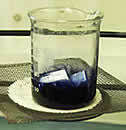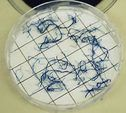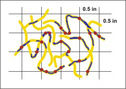# Plant & Mycorrhiza Root Lengths

In greenhouse experiments where all of the roots of an experimental plant can be collected and measured, then the grid-line intersect method (Giovannetti and Mosse, 1980) can be used to measure two important variables:

1. Mycorrhizal root length—an estimate of the length of roots colonized by mycorrhizal fungi (convertable to percentage colonization when divided by plant root length).
2. Plant root length—an estimate of the total length of roots produced by the plant.

## Calculating Plant and Mycorrhizal Root Length

Several small samples are removed from the main root mass after blotting, pooled, and fresh weight is measured (FW1). Total mass of this sample should be no more than 0.1-0.2 grams. ( The small root sample on the left will be used for one part of the testing while the several root sections on the right will be utilized in other testing.)

## Small Sample

Stain root sample in 0.05% direct blue or other acidic stain. Spread roots in plastic petri dish in which a grid with 0.5×0.5 squares is affixed to the base. Collect the following data:
(i) total number of intersects between lines and roots (R1)
(ii) total number of intersects where the root is mycorrhizal (R2)EXAMPLE: In the illustration at right,  R1 (root length) is 40 cm:
21 intersects (horizontal lines) + 19 intersects (vertical lines).

R2 (mycorrhizal root length) is 21 cm:
Intersects designated by red circles: 11 (horizontal) + 10 (vertical)
also: 21/40 = 53% colonization

## Other Samples

The remaining roots of the sample are weighed quickly (weight will decline rapidly as water evaporates) to obtain a measurement of fresh weight (FW2).

Measure dry weight of large sample (DW2).

## Total Sample

The data above is used to calculate plant root length and mycorrhizal root length of the total sample based on the assumption that distribution of mycorrhizae in the small pooled sample reflects the distribution of mycorrhizae throughout the entire root system.

Dry weight of small sample stained to measure colonization (DW1)

This calculation is performed by setting up a proportion and solving for x (DW1), as follows:

DW2/FW2 = x/FW1
(FW2)(x) = (FW1)(DW2)
x or DW1 = (FW1)(DW2)/FW2

Mycorrhizal root length in large (unstained) sample (R3)

This calculation is an estimate based on the actual measurement of mycorrhizal root length in the small stained sample (R2). Another proportion is set up and solved for x (R3), as follows:

R2/DW1 = x/DW2
(DW1)(x) = (R2)(DW2)
x or R3 = (R2)(DW2)/DW1

Total mycorrhizal root length = R2 + R3

Total plant root length can be estimated by substituting R1 for R2 in the same set of calculations. This estimate is valid only if the root architecture in the small sample (which usually consists of only fine roots) resembles that of the rest of the root system—the large sample.

## Reference

• Giovanetti, M. and B. Mosse. 1980. An evaluation of techniques for measuring vesicular mycorrhizal infection in roots. New Phytologist 97:447-453.